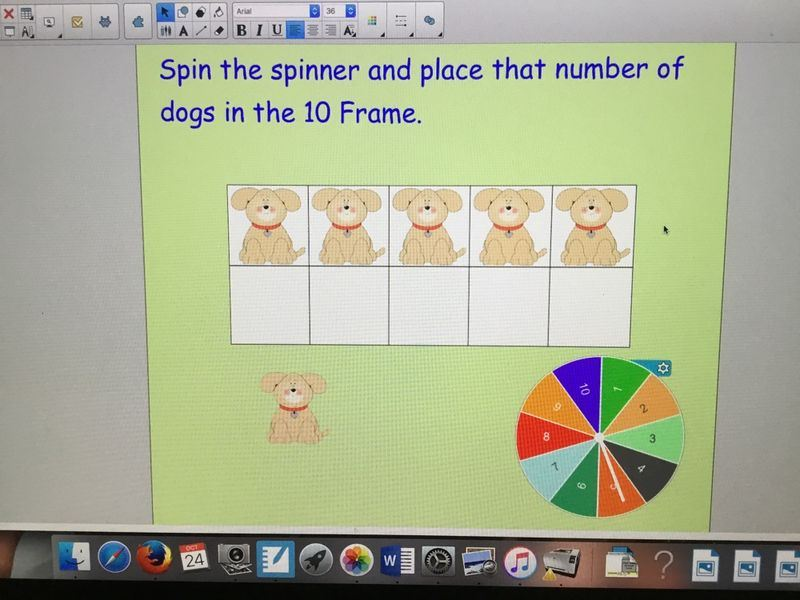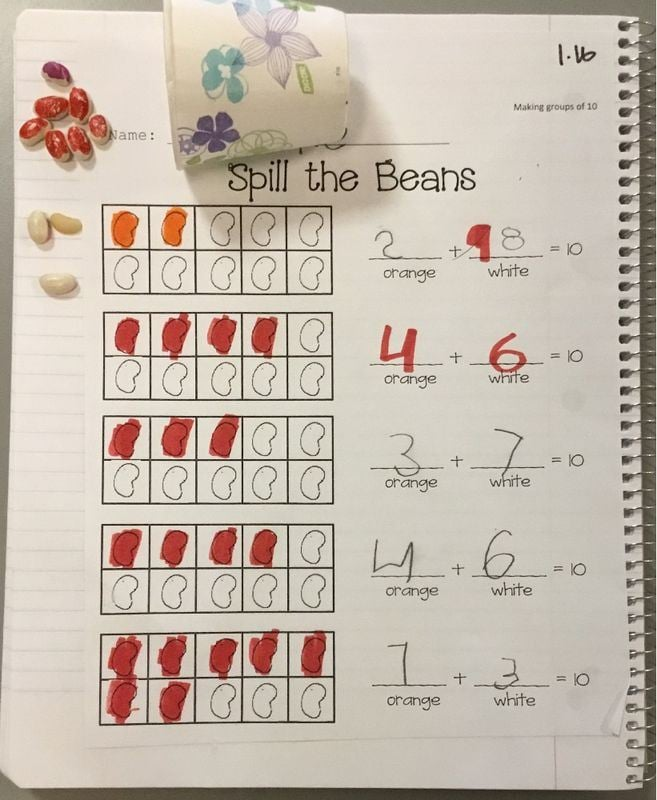# Terrific Ten Frame

In math this week, students have been exploring the ten frame. A ten frame is simply a graphic way for students to see numbers.This ten frame shows the number eight.

We started the lesson with some fun activities on the Smart Board where students had to build numbers less than ten.Then students had to look at a ten frame and identify the number of dogs in the frame.As an extension, I asked students if there were five dogs in the ten frame, how many empty spots were there? Five of course! This paved the way for our next activity that I like to call Spill the Beans. Each student received a cup with ten beans that were white on one side and orange on the other. They were to shake up the cup and spill out the beans, sorting them into groups according to color. Students then recorded how many orange beans they had and how many white beans they had.  They colored in the number of orange beans on the ten frame. Finally, students wrote and solved the addition equation.So what exactly does a ten frame teach? Ohhhh….so many concepts!

First, students learn number sense. It is through this number exploration that students learn the value of each number. For example, if numbers don’t fill one row, then the number is less than five. If the numbers fill more than the first row, the number is larger than five. Number sense is key to students’ success in advanced math.

Second, students look at numbers as sums including five. Students make the numbers to 10 and write them as composites of 5 and another number: i.e., 8= 5 + 3.

Third, students look at numbers in the context of ten. In other words, by using a ten frame, they figure out how many are needed to add to 6 to make ten. This will later help students decompose addition greater than ten: i.e., 8 plus 8 is 8 plus 2 plus 6, or 16.

In short, a ten frame can be used to build number sense, help students gain “mental math” fluency, and to better understand how to use the math strategies of “composing and decomposing” numbers, to complete operations over places (i.e., from tens to hundreds, or thousands to hundreds.) Go ten frame! Wait until you see the math centers I have planned for the students!